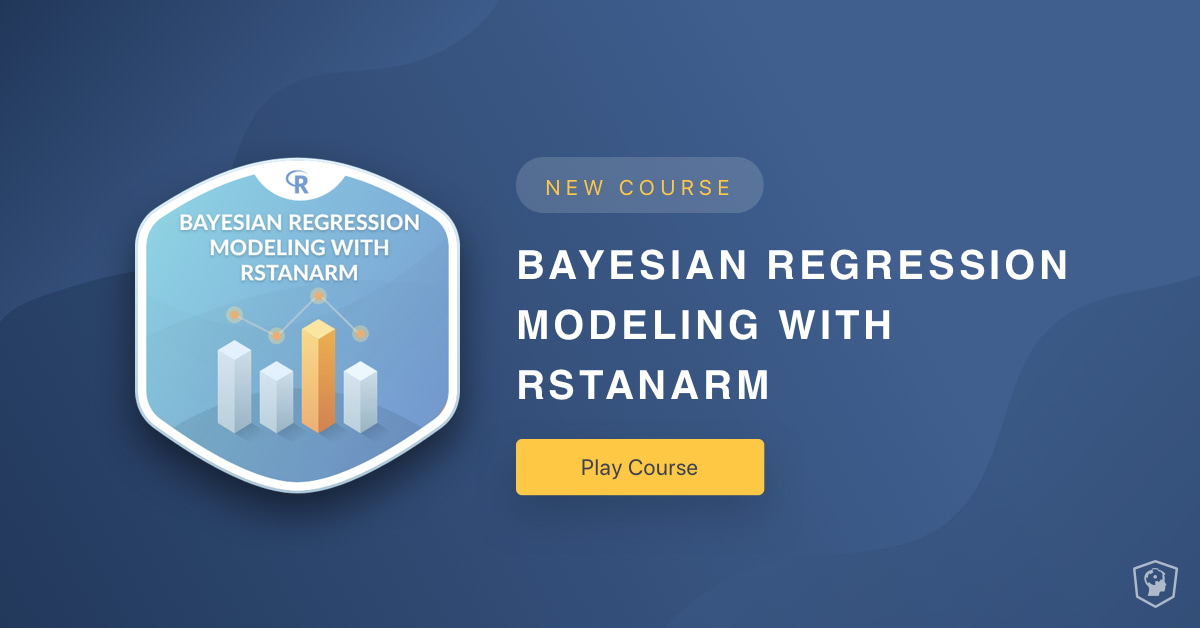# New Course: Bayesian Regression Modeling with rstanarm

Learn about our new R course.### Course Description

Bayesian estimation offers a flexible alternative to modeling techniques where the inferences depend on p-values. In this course, you’ll learn how to estimate linear regression models using Bayesian methods and the rstanarm package. You’ll be introduced to prior distributions, posterior predictive model checking, and model comparisons within the Bayesian framework. You’ll also learn how to use your estimated model to make predictions for new data.

### Chapter 1: Introduction to Bayesian Linear Models (Free)

A review of frequentist regression using lm(), an introduction to Bayesian regression using stan_glm(), and a comparison of the respective outputs.

### Chapter 2: Modifying a Bayesian Model

Learn how to modify your Bayesian model including changing the number and length of chains, changing prior distributions, and adding predictors.### Chapter 3: Assessing Model Fit

In this chapter, we'll learn how to determine if our estimated model fits our data and how to compare competing models.

### Chapter 4: Presenting and Using a Bayesian Regression

In this chapter, we'll learn how to use the estimated model to create visualizations of your model and make predictions for new data.